# Klein’s Quartic Equation

## by Greg Egan

Klein’s quartic curve is a surface of genus 3 (a three-holed torus) of constant negative curvature. It can be constructed by specifying a 14-gon in the hyperbolic plane and identifying pairs of edges. However, it can also be constructed as the solution to Klein’s wonderfully simple equation:

u3v + v3w + w3u = 0

You can read Klein’s derivation of this equation in his original article on the quartic curve, “On the Order-Seven Transformation of Elliptic Functions”, translated into English in this book:

The Eightfold Way: the Beauty of Klein’s Quartic Curve, edited by Silvio Levy; MSRI Research Publications 35, Cambridge University Press, Cambridge 1999.

It’s a wonderfully rich and dazzling piece of mathematics, definitely worth looking at even for readers like me who are unable to follow all of the details.

My purpose with this web page is to do something vastly simpler: to work backwards, starting from the quartic equation, and derive the topology and symmetries of its set of solutions. The algebra required to do this is much less heavy-duty than that employed in Klein’s paper.

## Topology

The first thing to note is that Klein’s quartic equation (KQE) is a homogeneous equation in three complex variables, u, v and w. If any triple of complex numbers, (u,v,w) solves the equation, then any multiple of that triple, (zu,zv,zw) for any complex number z, will also solve the equation.

What this means is that the solution space we’re really interested in is not C3, the vector space of ordered triples of complex numbers, but rather CP2, the two-complex-dimensional space that consists of all “rays” in C3, i.e. all one-complex-dimensional linear subspaces of C3. This space is known as the complex projective plane.

It’s not too hard to picture the complex projective plane. One helpful thing to do is to put a set of coordinates on it. Every ray in C3 will contain an element of the form:

(x exp(αi), y exp(βi), z)

where α and β are phase angles which lie between 0 and 2π, and x, y and z are non-negative real numbers such that (x,y,z) is a unit vector in R3. This tells us that most of CP2 can be thought of as the Cartesian product of the positive octant of a sphere in R3 (the x,y,z part of the coordinates, where of course only two of the coordinates are independent) and a torus (the α, β coordinates).

Be warned: this coordinate system is subject to the same kind of behaviour as any polar coordinate system. As well as the identification between the values 0 and 2π in the angular coordinates, there is singular behaviour whenever x, y or z becomes zero.

To get a clearer picture of the topology, suppose we fix the z coordinate, and allow x and y to vary along an arc of fixed latitude in the positive octant. The collection of tori generated as we move along the arc can be assembled into a 3-sphere.The picture above shows a 3-sphere divided in half and projected down to three dimensions. It has been foliated by tori, which appear here as cylinders of varying lengths and radii. The borders of the two parts should be identified, of course, and in fact we can join all the divided cylinders together to create a single piece.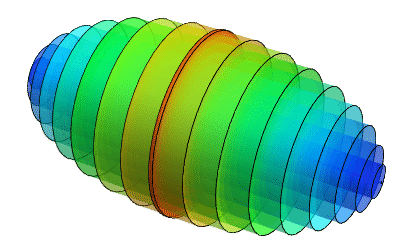We now only have to mentally join up the ends of these cylinders to form tori.

If we take α to be the coordinate that wraps around the cylinders, then β will range from 0 to 2π along their lengths. The radius of each cylinder is x and its length is 2πy. As we move along the arc of fixed latitude in the positive octant, we go from x=0, y=√(1–z2), which gives the circle formed by the straight line in this figure, to y=0, x=√(1–z2), which gives the circle that appears here as a circle.

So, an arc of fixed latitude on the positive octant corresponds to a 3-sphere. The size of the 3-sphere is determined by z, so we can vary our arc’s latitude to generate a family of 3-spheres of varying size. (Of course we haven’t put a metric on this space, so all talk of “size” is about coordinate values.) As z goes to 1, the whole 3-sphere shrinks to a single point. As z approaches zero, the 3-sphere reaches its maximum size. At first glance this might seem like a boundary to the manifold, but if we look at the representative point in C3 when z=0:

(x exp(αi), y exp(βi), 0)

we see that any two points with the same x and y coordinates and whose α and β coordinates differ by the same amount will now belong to the same complex ray — the one generated by (x, y exp((β–α)i), 0). So we need to mod out by this equivalence relation, and the tori all collapse into circles (while those that are already circles collapse into points). This turns the set where z=0 into a 2-sphere. This sudden change in dimension might seem odd, but the same kind of thing happens when, for example, you hit the centre of a polar coordinate system in the Euclidean plane and a 1-dimensional arc of a fixed angular size suddenly collapses to a point.

If either x or y is also equal to zero, the torus collapses to a single point. So the corners of the octant — (1,0,0), (0,1,0) and (0,0,1) — all represent single points in the whole space CP2.

Now, if we substitute the triple of complex numbers specified by our coordinates into KQE, it becomes:

exp((3α+β)i) x3y + exp(3βi) y3z + exp(αi) z3x = 0

We can simplify this version of the equation if we adopt a new pair of phase coordinates, γ and ψ, defined by:

γ = α – 3β
ψ = 3α – 2β

With these new coordinates, and dividing out an overall phase of 3β, we get:

exp(ψi) x3y + y3z + exp(γi) z3x = 0

By taking the real and imaginary parts of this equation, it’s fairly easy to find solutions for the ratios y/x and z/x. (For now, we will assume that x, y and z are all non-zero, along with various sines and cosines of phases; this will give us the bulk of the solution, and then we will come back and look more closely at the borderline cases.) The solutions are:

y/x = [ sin(γ–ψ)3 / (sin(γ)2 sin(ψ)) ]1/7
z/x = [ sin(ψ)2 sin(ψ–γ) / sin(γ)3 ]1/7

We require x, y and z to all be real and positive (we’re excluding zero for now), so these solutions will only be valid if the two quantities we’re taking the seventh roots of are both positive. This will occur in fourteen distinct domains in the (α, β) coordinates, separated by lines of the form γ=(2m+1)π, ψ=(2n+1)π, and γ–ψ=(2k+1)π, where m, n and k are integers: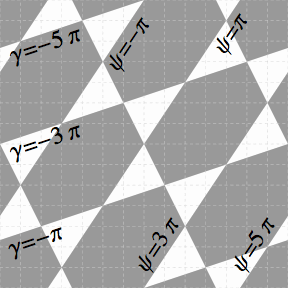We need to keep in mind that each (α,β) pair also has a location on the positive octant. The image of each domain on the octant can most easily be found by looking at the limiting behaviour of our solutions for y/x and z/x as we approach various parts of the border of each domain. It turns out that as we approach the three sides of the triangle in the torus, we approach three points on the octant, and as we approach the three vertices of the triangle in the torus, we approach three curves on the octant.

To see this, note that:

limγ→(2m+1)πx/z = limγ→(2m+1)πy/z = 0

so as we approach the border where γ=(2m+1)π, we must be approaching (0,0,1) in the octant. Similarly, as we approach the border where ψ=(2n+1)π, we approach (0,1,0), and as we approach the border where γ–ψ=(2k+1)π, we approach (1,0,0).

What happens when we approach one of the vertices of the triangular domains in the (α,β) torus? Clearly the map is not continuous on the vertex itelf, since the limit jumps abruptly from one point in the octant along one side to another point along another side. However, we can take a small line segment that truncates the corner and look at its limiting behaviour as it gets closer to the corner. This will give us a curve that lies on the border of the solution set projected on to the octant.

If we take the truncating line segment to be γ=ε, ψ=π+λε, for λ running from 0 to 1, then take the limit as ε goes to zero, we get:

limε→0y/x = (1–λ)3/7 / λ1/7
limε→0z/x = (1–λ)1/7 λ2/7

We can then solve for x, y and z in terms of λ, using the fact that these coordinates lie on the unit sphere. The results for the other vertices are similar, and we plot the results on the positive octant below. Here we have projected the octant on to an equilateral triangle in the plane, whose vertices are the projections of (1,0,0), (0,1,0) and (0,0,1).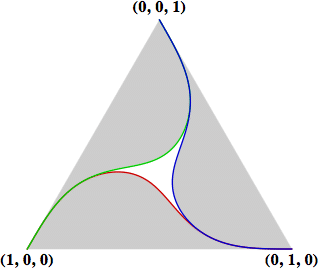For these three curves, we have:

 red curve (γ, ψ) = (0, π) modulo 2π –x3y + y3z + z3x = 0 green curve (γ, ψ) = (π, 0) modulo 2π x3y + y3z – z3x = 0 blue curve (γ, ψ) = (π, π) modulo 2π x3y – y3z + z3x = 0

where the equation in the third column can be found simply by substituting the values for γ and ψ into our version of KQE:

exp(ψi) x3y + y3z + exp(γi) z3x = 0

Now, once the x and y values that lie on these curves are multiplied by the appropriate phases, exp(αi) and exp(βi), we have solutions of KQE itself. These phases come from the vertices of the triangular domains in the torus coordinates, and there are a total of 21 such points from the 14 triangles. If we take the vertices (α, β) = (0, π), (π, 0) and (π, π), they are not shared by a single triangular domain, but their (γ, ψ) values are of each of the three kinds. The phases from these (α, β) values are just ±1, and taken together, the three curves that project to these vertices comprise the real-valued solutions of KQE.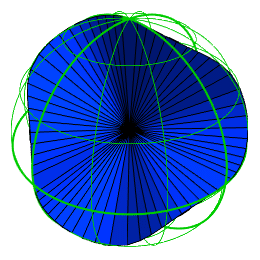The movie above shows the cone of rays in R3 for these solutions, and its intersection with the unit sphere. The two curves where the cone intersects the sphere become a single curve in the real projective plane RP2 (which can be thought of as the unit sphere with antipodal points identified), and similarly the whole set of real solutions is encompassed by a single one-dimensional curve in CP2.

We can get six more smooth, closed, one-dimensional curves in the solution space by repeatedly adding (6π/7, 2π/7) to the three (α, β) values for the real-valued solutions; this is equivalent to leaving γ constant and incrementing ψ by 2π. The picture below shows the resulting seven triples of (α, β) values, with vertices belonging to the same triple sharing the same colour.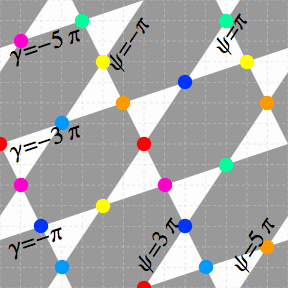In fact, the action of adding (6π/7, 2π/7) to (α, β) is a symmetry of the whole solution space. Since this action leaves γ unchanged and increases ψ by 2π, it will take any solution of KQE into another one. Another symmetry of the solution space is to cyclically permute u, v and w. In our coordinates, because we always cancel out the phase on the third complex number in the C3 triple, this is the map (x, y, z, α, β) → (z, x, y, –β, α–β). Between them, these order-3 and order-7 symmetries generate a 21-element subgroup of the symmetries of the solution space; the elements of this subgroup map triangular domains in the torus into each other. The single domain in the positive octant is always preserved as a set, but these symmetries can cyclically permute its vertices, (1,0,0), (0,1,0) and (0,0,1).

The picture of the solution space that we’ve assembled so far is that while the interior of each of the domains in the torus can be thought of as a generic projection of a piece of the solution from four dimensions down to two — and in each case the projection of this piece on to the octant is the interior of the region bounded by the same three curves — the borders of these pieces have non-generic projections. Three segments of the border each project down to single points on the torus: the vertices of the triangular domains. And three points on the border lie at coordinate singularities that make them look like lines in the torus coordinates: the edges of the triangular domains.

What’s more, for every triangular domain, the points in CP2 that the three sides of the triangle represent are the same three points for all 14 triangles. Every side that lies on a line of the form γ=(2m+1)π is really the single point (0,0,1); every side that lies on a line of the form ψ=(2n+1)π is really the single point (0,1,0), and every side that lies on a line of the form γ–ψ=(2k+1)π is really the single point (1,0,0).

It’s now clear that the surface at the vertex of each triangular domain must be twisted over the vertex like a ribbon turned 90 degrees. This means that alternating triangular domains will lie on opposite sides of the surface. In the picture below, the two sides have been coloured red and green. Note that this is just a stylised view illustrating the topology, it’s not any kind of rigorously computed projection of the solution set down to three dimensions.The view below shows the same surface from directly above, with edges that need to be identified marked in the same colour: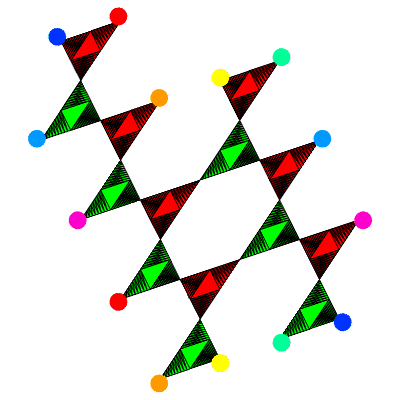We can untwist the connections between the domains with some judicious cutting, flipping and reconnecting, as shown below: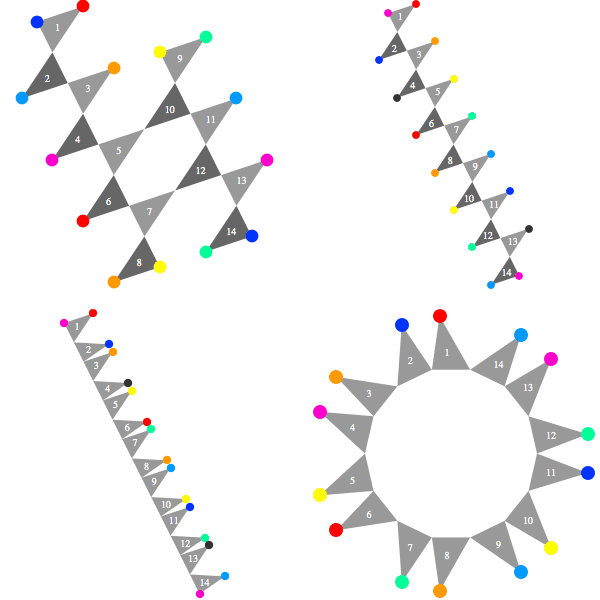Now we should replace these triangles with their duals: turning every edge into a vertex and every vertex into an edge. This reflects the true topology, since the edges in the torus coordinates are really single points, and the vertices in the torus coordinates are really 1-dimensional curves.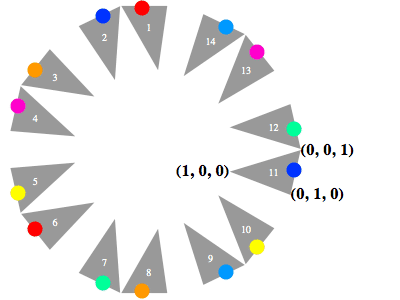The adjacent pairs of edges of the triangles should be taken to be identified, as well as the colour-coded identification of the edges on the outside of the ring. Also, all 14 of each set of similar vertices should be identified. One set of 14 vertices will obviously come together at the centre, but amazingly enough the identification of the edges achieves this for the other two sets as well.

The 14-gon we’ve created has its edges identified in exactly the same fashion as the 14-gon in the hyperbolic plane described in Klein’s original paper. So, we’ve successfully reconstructed the topology of Klein’s quartic curve from his quartic equation! We can verify that the surface has a genus of 3 by calculating its Euler characteristic; we have divided it into 14 triangles with 21 edges and just 3 vertices in total, so its Euler characteristic is FE+V=14–21+3=–4, which equals 2(1–g) for g=3.

Finally, here’s a slightly more quantitative map of some features of Klein’s quartic curve.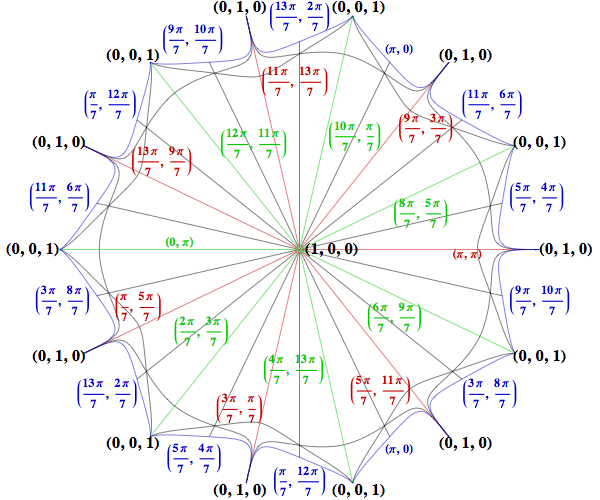The map above doesn’t follow the geometry of the hyperbolic plane, since we haven’t actually calculated anything involving the metric. Rather, the radial coordinate here is √(y2+z2) — so we have (1,0,0) in the centre and (0,1,0) and (0,0,1) at the perimeter of the unit circle — and the angular coordinate corresponds to a line sweeping across each domain in the torus coordinates, anchored at the blue vertex (see below left). The red, green and blue curves that border each segment are labelled by their (α, β) values. The three black lines that meet within each segment intersect at a point where x=y=z=1/√3. All of these curves are mapped on to the torus coordinates (below left) and the positive octant (below right); of course the coloured curves map to single points on the torus.

Where are the real-valued solutions on this map? They comprise the horizontal green and red lines that meet at the centre of the map, with phases (0, π) and (π, π), and the blue curve at the top of the map with phases (π, 0).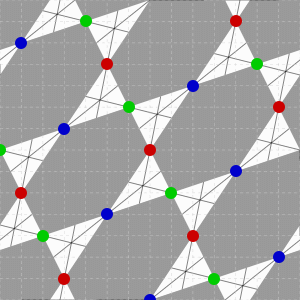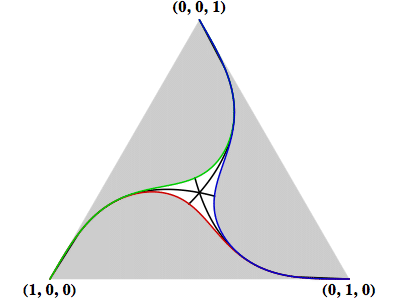## Symmetries

The purpose of this section is to derive the 168 symmetries of Klein’s quartic curve directly from the quartic equation itself. These symmetries are probably easiest to understand in terms of the Platonic tiling of the surface with 24 heptagons or 56 triangles, but there are some interesting features that arise by taking a simple algebraic approach.

### Symmetries of the torus

We’ve already mentioned the 21-element subgroup of symmetries which preserve as sets the 14 triangular domains in the torus coordinates. The translational symmetries are generated by the action of adding (6π/7, 2π/7) to (α, β). We will refer to this group element as S. This is an order-7 symmetry, and its repeated application can carry any triangular domain into any of the 6 others in the torus that are congruent to it (the 14 domains come in two shapes that are mirror images of each other). S has three fixed points: (x,y,z) = (1,0,0), (0,1,0) and (0,0,1).

The other element we need to generate the symmetries of the triangular domains is the rotation induced by cyclically permuting the three complex numbers (u,v,w). In our coordinates, this maps (x, y, z, α, β) to (z, x, y, –β, α–β). We will call this group element T. It has two fixed points, with x=y=z=1/√3 on the positive octant and (α, β) = (2π/3,4π/3) and (4π/3,2π/3). As vectors in C3 these are orthogonal.

Between them, S and T generate a 21-element subgroup, which can carry any domain into any congruent domain, while cyclically permuting the edges and vertices. The subgroup consists of the identity, the 6 translations of order 7 generated by S, and 14 elements of order 3. The order-3 elements each have two fixed points, lying at the centres of two domains. Pairs of domains associated with the same order-3 elements are coloured identically in the figure below.### Symmetries of the real solution cone

The cone of rays formed by the real solutions of KQE has three planes of mirror symmetry, illustrated below: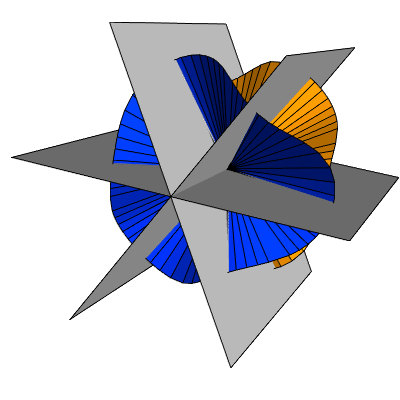We are not counting reflections among the 168 symmetries of Klein’s quartic curve, but we can still make use of these planes. Because we’re dealing with projective space, the distinction between linear operators with positive and negative determinants doesn’t apply; for example a reflection in the xy plane, which consists of reversing the z coordinate, has exactly the same effect on the set of rays as a rotation by 180 degrees around the z-axis, which reverses the x and y coordinates. The first transformation has a determinant of –1, the second of 1, but their action in projective space is identical. Reflections in CP2 involve a different operation: complex conjugation.

To get a clearer picture of the symmetries of the cone, we can shift to cylindrical polar coordinates (r,θ,z’), with the z’ axis parallel to the vector (1,1,1) in our original coordinates. We can then plot the curve of intersection of the cone with the plane z’=1.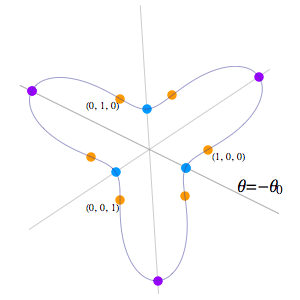The three planes of symmetry are drawn here as grey lines; the two kinds of points where they intersect the cone are marked in blue and violet. The six orange points are points of inflection; these include the intersections with the coordinate axes, as well as their three mirror images.

If we choose the projection of the +ve x-axis on to the plane as the angle where θ=0 then KQE becomes, in our new coordinates:

4z'4 + 6r2z'2 – 3r4 – (2√14) r3z' cos(3(θ+θ0)) = 0
θ0=(1/3) arccos(1/(2√7))

We will call the rotations by 180 degrees around the normals to each of the planes of symmetry R1, R2, and R3. These are order-2 elements. If we multiply two of these rotations around different axes, we get a rotation around (1,1,1), which is either T or T2. Together with the identity, they form a 6-element subgroup isomorphic to the dihedral group D3.

Although it appears that each Ri has just two fixed points, the blue and violet points on each plane of symmetry, in fact there are four fixed points. We can see this by setting cos(3(θ+θ0)) = 1 in the polar form of KQE above; this is the condition that identifies the planes of symmetry. The resulting quartic has two real solutions for r/z’, one positive and one negative, and also a conjugate pair of complex-valued solutions.

To reveal another kind of symmetry related to the real cone, we’ll need to look more closely at the intersection of the cone with one of the planes of symmetry.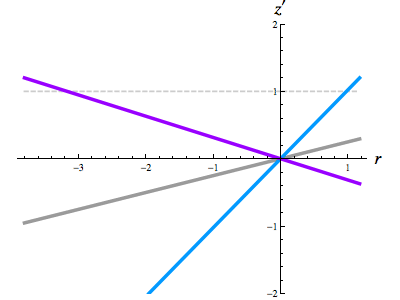The dashed grey line here is the plane z’=1 on which the previous figure was drawn; the solid grey line is a line of symmetry midway between the two real rays on the solution cone. We will choose a new set of Cartesian coordinates, (x'',y'',z''), such that the x'' axis coincides with the line of symmetry shown above, the y'' axis is orthogonal to the plane of the figure, and the z'' axis is orthogonal to both. In these coordinates, KQE becomes:

(√7) (x''4z''4) – 6x''2z''2 + 2y''4 - (12√2) x''y''2z'' = 0

First, we should note that we haven’t actually proved that our Ri preserve the quartic, but in these coordinates it’s easy. The Ri associated with whichever plane of symmetry we chose is a rotation by 180 degrees around the y'' axis, i.e. x'' → –x'', z'' → –z'', which clearly leaves all terms in the quartic unchanged.

Now, we would like to see if there is a transformation that exchanges the blue and violet rays while preserving the quartic. The most obvious candidate fails; rotating 180 degrees around the z'' axis, which amounts to negating both x'' and y'', does not leave the quartic invariant, thanks to the x''y''2z'' term. However, if we negate x'' while multiplying y'' by i, all terms are left unchanged. (If we wish to describe the same action with a transformation with determinant 1, we can multiply through by –i, so that x'' → i x'' and z'' → –i z''; the effect on the CP2 rays is the same.)

This symmetry has order 4, because it multiplies y'' by i, a fourth root of unity. As well as exchanging the blue and violet rays, it also swaps the two complex-valued points that lie on the same plane of symmetry. If we call this group element Qi, where the subscript i=1,2,3 identifies the plane of symmetry, then Qi2 will have order 2, and will amount to a rotation by 180 degrees around the y'' axis, so in fact it will just be Ri. The third power, Qi3, will have order 4.

### The 168 symmetries

We are now in a position to catalogue the 168 symmetries of Klein’s quartic curve.

First, we note that if we act on the real curve with S, S2, ..., S6, we end up with 7 curves that intersect each other only at the three points (1,0,0), (0,1,0) and (0,0,1). As well as the three points that they have in common, the 7 curves will contain 21 distinct inflection points, because the original curve also contained the “mirror” images of (1,0,0), (0,1,0) and (0,0,1), which can be found with any of the Ri. So in total we have 24 points of inflection, which we can group into 8 sets of 3.

Those 24 points correspond to the centres of the 24 heptagons of the Platonic tiling. We can associate 6 symmetries of order 7 with each of the sets of 3 points by conjugating S, S2, ..., S6 with the group elements which took (1,0,0), (0,1,0) and (0,0,1) to the three points. So we have identified a total of 48 symmetries of order 7.

Each of the 7 curves will also contain images of the 6 real fixed points of the order-2 elements R1, R2, and R3, and if we also copy the two complex fixed points of each Ri, there will be 12 points associated with each copy of the curve, making 84 points in all. The 84 points correspond to the centres of the 84 edges of the Platonic tiling. They break down into 21 sets of 4, and associated with each set is an order-2 element derived from one of the Ri, and two order-4 elements derived from Qi and Qi3.

To construct the fixed points of all the order-3 elements, we first need to choose a suitable order-4 element to replicate the fixed points of T. It turns out that Q'=SQ1S–1 serves our purpose. Repeated application of Q' to the fixed points of T yields 8 distinct points, and then repeated application of S yields a total of 56 points. The 56 points correspond to the 56 trivalent vertices of the heptagonal Platonic tiling, or the centres of the 56 triangles of the dual tiling. Conjugation of both T and T2 by the same powers of Q' and S will produce only 56 order-3 elements, since each order-3 element has two fixed points, and each fixed point belongs to two order-3 elements.

The 7 sets of 8 points produced by applying powers of S to the first set of 8 are each preserved by 7 different (but isomorphic) 24-element subgroups. These subgroups are all isomorphic to the symmetries of a cube (or octahedron) in R3. We can think of the two fixed points of T as two diagonally opposite vertices of a cube, and Q' as the 90-degree rotation around one of the faces of the cube, which generates all 8 vertices from one diagonal pair. (This picture can’t be taken literally for the points in C3, but it’s possible to map Klein’s quartic curve to R3 in a way that makes this correspondence exact.)

So, we have seen how to construct 48 symmetries of order 7, 21 symmetries of order 2, 42 symmetries of order 4, and 56 symmetries of order 3. Along with the identity element, that makes up the total of 168. If we also allow the possibility of composing each of these symmetries with complex conjugation of (u,v,w), which acts as a reflection in CP2, then there are a total of 336 symmetries.

The 168 symmetries can all be represented by elements of SU(3): complex unitary 3×3 matrices with determinant one, acting on rays in CP2 via their action on representative vectors in C3. (It will be necessary to multiply some of the obvious matrices by an overall factor to make their determinant one; for example, the two phases that S adds to α and β, 6π/7 and 2π/7, can be represented by a matrix with diagonal elements exp(8πi /7), exp(4πi /7), and exp(2πi /7).)

The fact that the symmetries are unitary means that all the fixed points we replicated by various symmetries will retain their orthogonality relationships. All 8 triples of fixed points of order-7 elements (the heptagon centres) will be mutually orthogonal, as will all 28 pairs of fixed points of order-3 elements (the triangle centres). The 21 sets of 4 fixed points of order-2 elements (the edge centres) are not orthogonal, they are coplanar.

### The fixed points

Since the various fixed points of the symmetries that we have catalogued constitute the major landmarks on Klein’s quartic curve, it’s worth summarising their locations.

We have already seen that the real curve contains 6 heptagon centres (that is, fixed points of order-7 symmetries), and the seven images of the real curve under powers of S contain all 24 heptagon centres. In the torus coordinates, the real curve and its images under powers of S make up the 21 vertices of the triangular domains; 21 of the heptagon centres lie on these vertices, while the three fixed points of S itself, (0,0,1), (0,1,0) and (0,0,1) are the edges of the triangular domains.

We noted earlier that the 14 centres of the triangular domains are all fixed points of order-3 symmetries. There are a total of 56 order-3 fixed points, so where are the other 42? It turns out that they all lie on the real curve and its copies.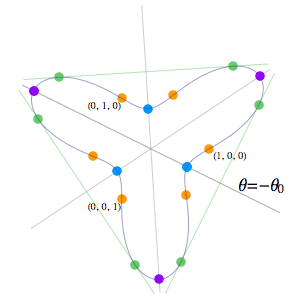The six green points in this picture of the real curve are all fixed points of order-3 symmetries. They come in three pairs which belong to orthogonal rays, and are fixed points of the same two symmetries. The pairs also belong to shared tangents to the curve; if you read Klein’s paper, this is what all the talk of “bitangents” is about. Although we can identify these points on the real curve, the symmetries of which they are fixed points are not symmetries of the real curve itself; as linear operators on C3, they multiply the two vectors of each pair by different complex eigenvalues.

So, the order-3 fixed points are either at the centres of the triangular domains of the torus coordinates, or at the vertices.

That leaves the fixed points of the order-2 symmetries. There are six on each copy of the real curve, which accounts for 42 of them, but there are also six more “associated with” the real curve that come from the complex-valued solutions of the quartic on the planes of symmetry. There is no simple characterisation of the phases of these points and their images under powers of S, but by symmetry it’s clear that three lie in the interiors of each of the 14 triangular domains of the torus coordinates.

### The 28 images of the real curve

We used the 7 images of the real curve under powers of S to help enumerate the symmetries of Klein’s quartic curve. Now that we have obtained all those other symmetries, we can look at what they do to the real curve.

There is a 6-element subgroup that preserves the real curve as a set, so it follows that there will be a total of 168/6=28 different images of the real curve. Of course these curves will not be disjoint. The real curve contains two triples of order-7 fixed points, so there will be 2*(7–1)=12 curves that share three points with it. It also contains three pairs of order-3 fixed points, so there will be 3*(3–1)=6 curves that share two points with it. The order-2 Ri symmetries with fixed points on the real curve preserve the whole curve as a set, so they don’t generate new copies. However, the order-4 symmetries Qi that swap pairs of fixed points of the Ri involve imaginary factors, so they can’t preserve the real curve. Each Qi will generate a new curve, for i=1,2,3, but the other order-4 symmetry, Qi3, will generate the same curve, because Qi2=Ri will preserve the new curve generated by Qi. This means there will be a further 3 curves that intersect the real curve at two points, along with the 6 from the order-3 fixed points.

In total then, every one of these 28 curves will share 3 points with 12 others, and 2 points with 9 others, while being disjoint from 6 others.

All the fixed points lie on these curves at points of intersection. Each of the 28 curves contains 6 order-7 fixed points, lying at the intersection of 7 curves, giving a total of (28*6)/7=24. Each curve also contains 6 order-3 fixed points, lying at the intersection of 3 curves, giving a total of (28*6)/3=56. And each curve contains 6 order-2 fixed points, lying at the intersection of 2 curves (both individually preserved as sets by the order-2 symmetry), giving a total of (28*6)/2=84.

## Visualising the full solution

Although we have described the topology of the solution to Klein’s quartic equation, we don’t yet have a clear picture of the full solution as it sits in C3 or CP2. There are many different ways of visualising the solution; the following is just one possibility.

If we make the substitution (u,v,w) = (x exp(αi), y exp(–2αi), 1), KQE becomes:

x3y + exp(–7αi) y3 + x = 0

This has a manifest order-7 symmetry, α → α+2π/7. This is equivalent to the order-7 symmetry S5.

For a given value of α, we can plot the three solutions of this cubic in y for a range of real values of x; generically y will be complex-valued, so this amounts to taking a three-real-dimensional slice through the solution, with a basis parameterised by α. If we make a movie for values of α ranging from 0 to 2π, the three-dimensional slice will sweep across the entire solution. In fact, if we include negative values for x, then every point will be seen by the time α reaches π, since multiplication by –1 is the same as adding π to the phase. What’s more, we only need to calculate frames of the movie for α ranging from 0 to 2π/7, because the view repeats itself exactly, seven times in each full cycle of 2π.

To simplify the algebra we have chosen to work with vectors on the plane w=1, but that condition requires u and v to become increasingly large as we approach rays parallel to the plane. We will rescale the solutions by 1/|(u,v,w)| before displaying them, effectively changing the representative of each ray to one where w is real and |(u,v,w)|=1. The resulting three-dimensional slice is then bounded by the sphere x2+(Re y)2+(Im y)2=1. On the surface of the sphere, w=0, so in general antipodal points should be identified, while the whole circle x=0, |y|=1 belongs to a single ray.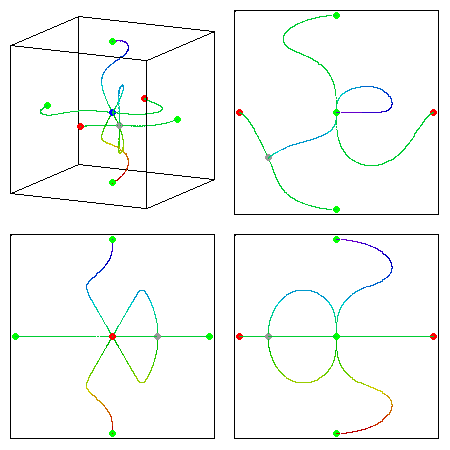As well as the three-dimensional view at the top left (where Im y is plotted vertically), three orthogonal projections are shown, like the plan and elevation views in an architectural drawing. The colour of the solution curves depends on Im y, with red (negative) shading to green (close to zero) then blue (positive). The fixed points of S, (1,0,0), (0,1,0) and (0,0,1) appear as red, green and blue dots; the two red dots should be taken as the same point, as should the four green dots.

Apart from these points, the solution curves intersect twice in each cycle, at α=0 and α=π/7; the points of intersection are marked with grey dots in the frames shown below. At α=0 (below left), the real curve appears in green; in subsequent cycles the view is repeated, but the same green curve represents, in turn, each of the 7 different curves produced from the real curve by applying powers of S.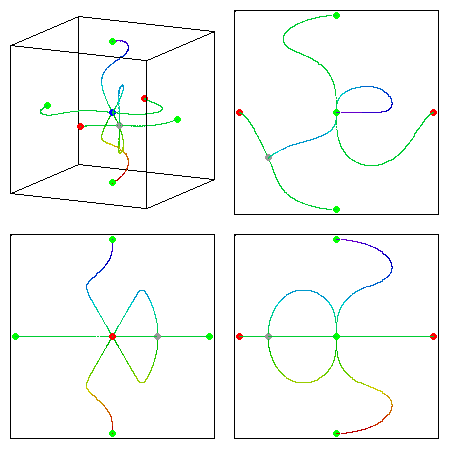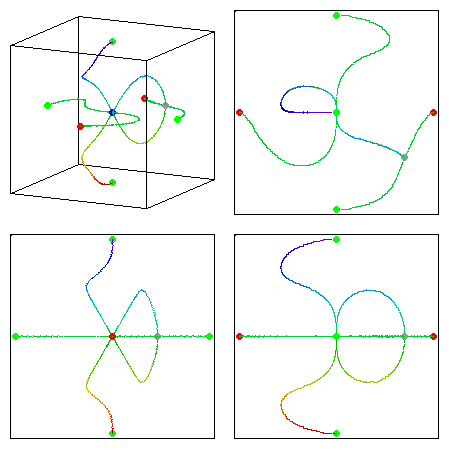α=0 α=π/7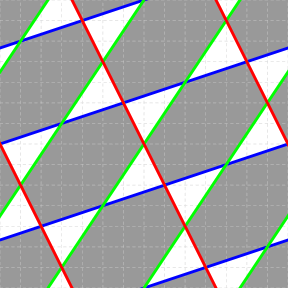We can relate this movie to our orginal torus and octant coordinates as follows. A line through the torus at a generic value of α will intersect three of the triangular domains along three line segments; these correspond to the three solution curves of the cubic. If we project the positive octant down to the positive xy quadrant, and then spin it around the x-axis to encompass different values for the phase β, we get a hemisphere, which is one half of the view in any frame of this movie. Because we are including negative x, we need to supplement this with a second line through the torus at α+π, and a second hemisphere.

At values of α equal to multiples of π/7 (the vertical broken lines in the figure on the left), the lines through the torus coordinates will pass through vertices of the triangular domains. These vertices themselves comprise entire curves (portions of the real curve and its copies), and together each pair of lines at α and α+π will pass through two triangular domains from edge to edge (without touching any vertices), two vertices cleanly (without touching any edges), and a pair of triangular domains from the edge of one to the parallel edge of the other (two blue edges, in the figure on the left), while passing through a vertex on its way. The line segment that joins two parallel edges gives rise to the loop from the blue dot (0,0,1) back to itself in the two frames of the movie shown above, intersecting a second curve, which comes from the vertex, joining the red dot (1,0,0) to the green dot (0,1,0).

## Parameterising the solution

Although our description of the solution set in terms of the torus coordinates allowed us to determine its topology, its behaviour over the 14 triangular domains is rather messy to deal with, especially given the fact that 1-dimensional curves sit over the 21 vertices of the domains. More convenient parameterisations are possible, and we will give one simple example.

We’ll define the following functions of a complex variable s:

A(s) = (1 + s7)1/7
B(s) = (1 – s7)1/7
Q(s) = (A(s)3 B(s), 23/7 A(s) s3, –21/7 B(s)3 s)

If we make the substitution (u,v,w)=Q(s), then the quartic form becomes:

u3v + v3w + w3u
= 23/7 A(s)10 B(s)3 s3 – 210/7 A(s)3 B(s)3 s10 – 23/7 A(s)3 B(s)10 s3
= 23/7 A(s)3 B(s)3 s3 [A(s)7 – 2s7B(s)7]
= 0

In other words, Q(s) satisfies KQE for all values of the complex variable s.

If we set s to zero; or to the zeroes of B(s) (which are the seventh roots of 1); or to the zeroes of A(s) (which are the seventh roots of –1), we will get values of Q(s) where two coordinates are zero:

Q(0) ~ (1,0,0)
Q(11/7) = Q(exp(2kπi /7)) ~ (0,1,0), k an integer
Q((–1)1/7) = Q(exp((2k+1)πi /7)) ~ (0,0,1), k an integer

If we choose the usual branch cuts along the -ve half of the real axis for the seventh roots in our definitions of A(s) and B(s), then Q(s) has the following branch cut structure in its domain: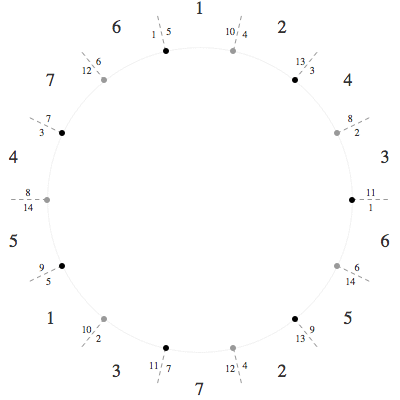Here the black dots are seventh roots of 1, the grey dots are seventh roots of –1, and the dashed lines are branch cuts which extend to infinity. The edges on each side of the branch cut are numbered 1–14; we can remove the discontinuities in Q(s) here by identifying edges with the same numbers. The larger numbers, 1–7, indicate seven distinct limits at infinity for Q(s) when the limit is taken between the pairs of branch cuts.

What values of s map to the real curve? If s is real and |s|<1, or if s lies on the edges numbered 4 or 13, then Q(s) is real.

We can treat this figure as a 28-gon, with 9 distinct vertices: one vertex from the seventh roots of 1, another from the seventh roots of –1, and 7 vertices at infinity. The identifications along the branch cuts mean that there are just 14 edges. The Euler characteristic is FE+V=1–14+9=–4, as we expect for a surface of genus 3.